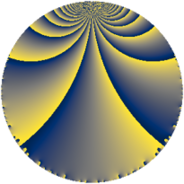# Properties

 Label 6552.2.a.lLevel $6552$ Weight $2$ Character orbit 6552.a Self dual yes Analytic conductor $52.318$ Analytic rank $0$ Dimension $1$ CM no Inner twists $1$

# Related objects

Show commands: Magma / PariGP / SageMath

## Newspace parameters

comment: Compute space of new eigenforms

[N,k,chi] = [6552,2,Mod(1,6552)]

mf = mfinit([N,k,chi],0)

lf = mfeigenbasis(mf)

from sage.modular.dirichlet import DirichletCharacter

H = DirichletGroup(6552, base_ring=CyclotomicField(2))

chi = DirichletCharacter(H, H._module([0, 0, 0, 0, 0]))

N = Newforms(chi, 2, names="a")

//Please install CHIMP (https://github.com/edgarcosta/CHIMP) if you want to run this code

chi := DirichletCharacter("6552.1");

S:= CuspForms(chi, 2);

N := Newforms(S);

 Level: $$N$$ $$=$$ $$6552 = 2^{3} \cdot 3^{2} \cdot 7 \cdot 13$$ Weight: $$k$$ $$=$$ $$2$$ Character orbit: $$[\chi]$$ $$=$$ 6552.a (trivial)

## Newform invariants

comment: select newform

sage: f = N # Warning: the index may be different

gp: f = lf \\ Warning: the index may be different

 Self dual: yes Analytic conductor: $$52.3179834043$$ Analytic rank: $$0$$ Dimension: $$1$$ Coefficient field: $$\mathbb{Q}$$ Coefficient ring: $$\mathbb{Z}$$ Coefficient ring index: $$1$$ Twist minimal: no (minimal twist has level 2184) Fricke sign: $$-1$$ Sato-Tate group: $\mathrm{SU}(2)$

## $q$-expansion

comment: q-expansion

sage: f.q_expansion() # note that sage often uses an isomorphic number field

gp: mfcoefs(f, 20)

 $$f(q)$$ $$=$$ $$q - q^{7}+O(q^{10})$$ q - q^7 $$q - q^{7} + 2 q^{11} - q^{13} + 2 q^{17} + 8 q^{23} - 5 q^{25} - 2 q^{29} - 4 q^{31} + 6 q^{37} + 8 q^{41} - 4 q^{43} - 6 q^{47} + q^{49} + 2 q^{53} + 2 q^{59} + 2 q^{61} - 4 q^{67} + 6 q^{71} - 2 q^{73} - 2 q^{77} + 6 q^{83} - 4 q^{89} + q^{91} - 2 q^{97}+O(q^{100})$$ q - q^7 + 2 * q^11 - q^13 + 2 * q^17 + 8 * q^23 - 5 * q^25 - 2 * q^29 - 4 * q^31 + 6 * q^37 + 8 * q^41 - 4 * q^43 - 6 * q^47 + q^49 + 2 * q^53 + 2 * q^59 + 2 * q^61 - 4 * q^67 + 6 * q^71 - 2 * q^73 - 2 * q^77 + 6 * q^83 - 4 * q^89 + q^91 - 2 * q^97

## Embeddings

For each embedding $$\iota_m$$ of the coefficient field, the values $$\iota_m(a_n)$$ are shown below.

For more information on an embedded modular form you can click on its label.

comment: embeddings in the coefficient field

gp: mfembed(f)

Label $$\iota_m(\nu)$$ $$a_{2}$$ $$a_{3}$$ $$a_{4}$$ $$a_{5}$$ $$a_{6}$$ $$a_{7}$$ $$a_{8}$$ $$a_{9}$$ $$a_{10}$$
1.1
 0
0 0 0 0 0 −1.00000 0 0 0
 $$n$$: e.g. 2-40 or 990-1000 Significant digits: Format: Complex embeddings Normalized embeddings Satake parameters Satake angles

## Atkin-Lehner signs

$$p$$ Sign
$$2$$ $$1$$
$$3$$ $$-1$$
$$7$$ $$1$$
$$13$$ $$1$$

## Inner twists

This newform does not admit any (nontrivial) inner twists.

## Twists

By twisting character orbit
Char Parity Ord Mult Type Twist Min Dim
1.a even 1 1 trivial 6552.2.a.l 1
3.b odd 2 1 2184.2.a.k 1
12.b even 2 1 4368.2.a.g 1

By twisted newform orbit
Twist Min Dim Char Parity Ord Mult Type
2184.2.a.k 1 3.b odd 2 1
4368.2.a.g 1 12.b even 2 1
6552.2.a.l 1 1.a even 1 1 trivial

## Hecke kernels

This newform subspace can be constructed as the intersection of the kernels of the following linear operators acting on $$S_{2}^{\mathrm{new}}(\Gamma_0(6552))$$:

 $$T_{5}$$ T5 $$T_{11} - 2$$ T11 - 2 $$T_{17} - 2$$ T17 - 2

## Hecke characteristic polynomials

$p$ $F_p(T)$
$2$ $$T$$
$3$ $$T$$
$5$ $$T$$
$7$ $$T + 1$$
$11$ $$T - 2$$
$13$ $$T + 1$$
$17$ $$T - 2$$
$19$ $$T$$
$23$ $$T - 8$$
$29$ $$T + 2$$
$31$ $$T + 4$$
$37$ $$T - 6$$
$41$ $$T - 8$$
$43$ $$T + 4$$
$47$ $$T + 6$$
$53$ $$T - 2$$
$59$ $$T - 2$$
$61$ $$T - 2$$
$67$ $$T + 4$$
$71$ $$T - 6$$
$73$ $$T + 2$$
$79$ $$T$$
$83$ $$T - 6$$
$89$ $$T + 4$$
$97$ $$T + 2$$